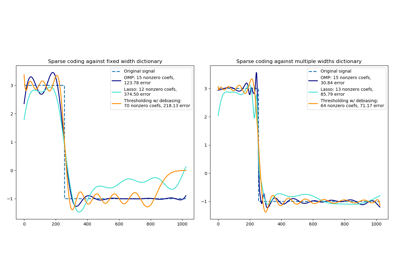# sklearn.decomposition.SparseCoder¶

class sklearn.decomposition.SparseCoder(dictionary, transform_algorithm='omp', transform_n_nonzero_coefs=None, transform_alpha=None, split_sign=False, n_jobs=None, positive_code=False, transform_max_iter=1000)[source]

Sparse coding

Finds a sparse representation of data against a fixed, precomputed dictionary.

Each row of the result is the solution to a sparse coding problem. The goal is to find a sparse array code such that:

X ~= code * dictionary


Read more in the User Guide.

Parameters
dictionaryarray, [n_components, n_features]

The dictionary atoms used for sparse coding. Lines are assumed to be normalized to unit norm.

transform_algorithm{‘lasso_lars’, ‘lasso_cd’, ‘lars’, ‘omp’, ‘threshold’}, default=’omp’

Algorithm used to transform the data: lars: uses the least angle regression method (linear_model.lars_path) lasso_lars: uses Lars to compute the Lasso solution lasso_cd: uses the coordinate descent method to compute the Lasso solution (linear_model.Lasso). lasso_lars will be faster if the estimated components are sparse. omp: uses orthogonal matching pursuit to estimate the sparse solution threshold: squashes to zero all coefficients less than alpha from the projection dictionary * X'

transform_n_nonzero_coefsint, default=0.1*n_features

Number of nonzero coefficients to target in each column of the solution. This is only used by algorithm='lars' and algorithm='omp' and is overridden by alpha in the omp case.

transform_alphafloat, default=1.

If algorithm='lasso_lars' or algorithm='lasso_cd', alpha is the penalty applied to the L1 norm. If algorithm='threshold', alpha is the absolute value of the threshold below which coefficients will be squashed to zero. If algorithm='omp', alpha is the tolerance parameter: the value of the reconstruction error targeted. In this case, it overrides n_nonzero_coefs.

split_signbool, default=False

Whether to split the sparse feature vector into the concatenation of its negative part and its positive part. This can improve the performance of downstream classifiers.

n_jobsint or None, default=None

Number of parallel jobs to run. None means 1 unless in a joblib.parallel_backend context. -1 means using all processors. See Glossary for more details.

positive_codebool, default=False

Whether to enforce positivity when finding the code.

New in version 0.20.

transform_max_iterint, default=1000

Maximum number of iterations to perform if algorithm='lasso_cd' or lasso_lars.

New in version 0.22.

Attributes
components_array, [n_components, n_features]

The unchanged dictionary atoms

Methods

 fit(self, X[, y]) Do nothing and return the estimator unchanged fit_transform(self, X[, y]) Fit to data, then transform it. get_params(self[, deep]) Get parameters for this estimator. set_params(self, \*\*params) Set the parameters of this estimator. transform(self, X) Encode the data as a sparse combination of the dictionary atoms.
__init__(self, dictionary, transform_algorithm='omp', transform_n_nonzero_coefs=None, transform_alpha=None, split_sign=False, n_jobs=None, positive_code=False, transform_max_iter=1000)[source]

Initialize self. See help(type(self)) for accurate signature.

fit(self, X, y=None)[source]

Do nothing and return the estimator unchanged

This method is just there to implement the usual API and hence work in pipelines.

Parameters
XIgnored
yIgnored
Returns
selfobject

Returns the object itself

fit_transform(self, X, y=None, **fit_params)[source]

Fit to data, then transform it.

Fits transformer to X and y with optional parameters fit_params and returns a transformed version of X.

Parameters
Xnumpy array of shape [n_samples, n_features]

Training set.

ynumpy array of shape [n_samples]

Target values.

**fit_paramsdict

Returns
X_newnumpy array of shape [n_samples, n_features_new]

Transformed array.

get_params(self, deep=True)[source]

Get parameters for this estimator.

Parameters
deepbool, default=True

If True, will return the parameters for this estimator and contained subobjects that are estimators.

Returns
paramsmapping of string to any

Parameter names mapped to their values.

set_params(self, **params)[source]

Set the parameters of this estimator.

The method works on simple estimators as well as on nested objects (such as pipelines). The latter have parameters of the form <component>__<parameter> so that it’s possible to update each component of a nested object.

Parameters
**paramsdict

Estimator parameters.

Returns
selfobject

Estimator instance.

transform(self, X)[source]

Encode the data as a sparse combination of the dictionary atoms.

Coding method is determined by the object parameter transform_algorithm.

Parameters
Xarray of shape (n_samples, n_features)

Test data to be transformed, must have the same number of features as the data used to train the model.

Returns
X_newarray, shape (n_samples, n_components)

Transformed data

## Examples using sklearn.decomposition.SparseCoder¶